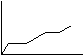Summer Research Program for Science Teachers

Partners in Science

Gregory Linn

High School of Math, Science and Engineering at CCNY

Manhattan

August 2004

Teaching the concept of chemical potential energy

Ninety-minute chemistry lesson.

Current thought regarding the best way to teach science has evolved to where it is felt by many that the current 45 or 50 minute class period is not sufficient time to include the types of teaching techniques needed to effectively teach students.

The feeling is that the simple chalk and talk method cannot reach all students given that students learn in different ways.  Some can easily handle copying notes from the board and assimilating the information later on.  Others who are poor note takers or simply do not study well do not fare well in this system.  Further, resent research suggests that students loose their concentration in a lecture after 20 to 30 minutes of lecture thus the remaining time is better spent on reinforcing the first half of the class using other techniques.

This need to go beyond the chalk and talk method is even clearer using 90 minute block classes.  Clearly, one cannot lecture for ninety minutes.  Thus a new approach to teaching must be developed.

Student elicited aim 10 minutes

The goal is to have students elicit the aim by starting off with examples of mechanical potential energy (MPE).

Using a drawing of a ledge some height above the ground and a ball resting on it, have students discuss amongst themselves the factors that influence the MPE-mass of ball, its heights and force of gravity.  Derive the equation for MPE: PE = mgh.

Then ask them if one talks about MPE in chemical reactions?  Are chemicals influenced by m, g, h?  Answer: No

Then ask students to come up with an aim based on this discussion.  It should be “what are the factors that affect chemical potential energy (CPE)?”

Lecture:

Lecture for about 20-30 minutes on chemical potential energy.

DCPE depends on                                                        DMPE depends on

a. mass                                                                         a. mass

b. nature of chemical-specific heat.                                b. nature of gravity

c. change in temperature                                               c. change in heights

Write formula for DCPE: P.E. = m∆T x spc. heat

What are the similarities between DCPE and MPE?

a. each involves a constant mass

b. each involves a constant (gravity or specific heat)

c. each involves a variable (height or DT)  Hence DT is analogous to height!

Problem solving: 20 minutes

Hand out exercises related to DCPE.  Problems included should concern students being able to understand the difference between temperature and heat content (DCPE).  Calculate the DCPE when raising the temperature of 100 g water from 25KC-50KC and same for 1000 g water.  Heat contents are different.  Go around to each student to make sure they are doing problems correctly.

# Elicite d aim

As before, start lesson with general questions regarding the nature of energy and heat.

For example: What is the difference between chemical potential and chemical kinetic energy? How can one experimentally change either chemical potential or kinetic energy?

How can one represent these changes?

Lecture 30 minutes

Heating curves are a great way for students to understand the connection between temperature and kinetic energy.  Take an ice cube and stick a thermometer inside it.  Or better yet, freeze thermometer in an ice cube. Start by asking students what happens to an ice cube as it begins to absorb heat from the environment.  Answer: the temperature increases while the cube is still solid.  When the cube melts completely, the temperature stops rising.  Kinetic energy is a measure of temperature and potential energy is a measure of distance between objects.  Thus since water molecules in ice have separated to become liquid, potential energy has changed..  Ask students what would happen if the liquid water was heated until boiling.  Draw a heating like the one below to illustrate the phase/energy changes of water.Demonstration/Laboratory

You can demonstrate the making of a heating curve by filling a test tube about one third the way up with water.  Place a thermometer into the test tube but not touching the bottom.  Immerse the filled test tube in a ice/salt mixture until the water is frozen.  Remove the test tube and place it in a test tube rack.  Record the temperature every thirty seconds until the water is completely melted.  Graph the results.  The curve should look like the first two lines of the graph illustrated above.  To obtain the rest of the curve,  start with the frozen water as before and heat the sample using a heated water bath.  Record the temperature until the water in the test tube begins to boil.  Have students graph the results and let them choose the labels for the axes.

You will not have enough time to finish this lesson so you may wish to stop before the demonstration.  Start it at the beginning of the next lesson.

National Standards

NS.9.12.1 Content Standard A; in this inquiry based approach, students are encouraged to begin asking questions about the factors that affect chemical potential energy.  From answering this question students can begin to focus on how to test these factors.

NS.9.12.2 Content Standard A; the lesson focuses directly on the nature of potential energy and the different phases of matter as they relate to energy. Students have learned the relation between the macroscopic concept of heat and temperature and the microscopic forces between molecules that are responsible for the manifestation of temperature changes.

New York State Standards.

Standard A: This will in part be achieved by the lab experience in that students will design

Standard B:  Mastery of concepts gained from combination of lecture, group work and text assignments

Standard E:  Use of lab equipment and how they are used to measure/detect things